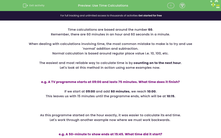# Use Time Calculations

In this worksheet, students will practise counting forwards to calculate end times, backwards to calculate start times or find the difference between two times using calculations based around 60.Key stage:  KS 4

Year:  GCSE

GCSE Subjects:   Maths

GCSE Boards:   AQA, Eduqas, Pearson Edexcel, OCR,

Popular topics:   Geometry worksheets

Difficulty level:#### Worksheet Overview

Time calculations are based around the number 60.

Remember, there are 60 minutes in an hour and 60 seconds in a minute.

When dealing with calculations involving time, the most common mistake to make is to try and use 'normal' addition and subtraction.

Normal calculation is based around regular place value i.e. 10, 100, etc.

The easiest and most reliable way to calculate time is by counting on to the next hour.

Let's look at this method in action using some examples now.

e.g. A TV programme starts at 09:00 and lasts 75 minutes. What time does it finish?

If we start at 09:00 and add 60 minutes, we reach 10:00.

This leaves us with 15 minutes until the programme ends, which will be at 10:15.

As this programme started on the hour exactly, it was easier to calculate its end time.

Let's work through another example now where we must work backwards.

e.g. A 50-minute tv show ends at 15:45. What time did it start?

For this one, we're going backwards so we need to count down.

From 15:45 to 15:00, we have used 45 minutes.

We just need to take an extra 5 minutes off.

This means the programme must have started at 14:55.

e.g. The winner of a formula one race has a finishing time of 1 hr 58 minutes. If the second-place finisher has a time of 2 hrs 4 minutes, how long after the winner did the 2nd place driver finish?

This is easier than it looks, as all we want to know is the difference between 1 hr 58 mins and 2 hrs 4 mins.

If we count on to the next full hour from 1 hr 58 mins, it takes 2 minutes to reach 2 hours.

We can then add 4 minutes more to arrive at 2 hrs 4 minutes.

This means that the total difference between the two times is 6 minutes.

In this activity, we will count forwards to calculate end times, backwards to calculate start times or find the difference between two times, all using calculations based around the number 60.

### What is EdPlace?

We're your National Curriculum aligned online education content provider helping each child succeed in English, maths and science from year 1 to GCSE. With an EdPlace account you’ll be able to track and measure progress, helping each child achieve their best. We build confidence and attainment by personalising each child’s learning at a level that suits them.

Get started